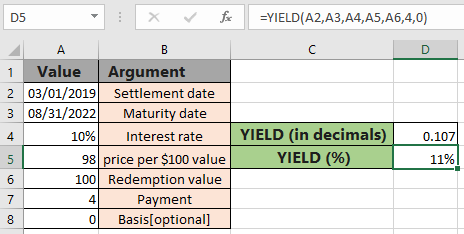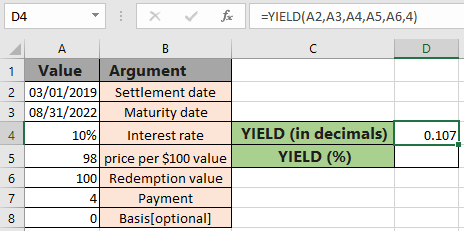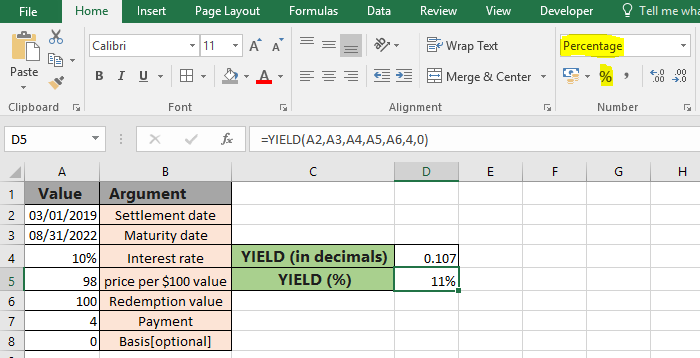# How to use the YIELD function in ExcelIn this article, we will learn How to use the YIELD function in Excel.

Scenario:

When working with the deposited security or bond value. Sometimes we need to find the Yield interest that shows how much income has been returned from an investment based on initial security, but it does not include capital gains in its calculation. Basically yield on a security that pays periodic interest. The yield is usually expressed as an annual percentage rate based on the investment's cost, current market value, or face value.

YIELD function in Excel

YIELD function is excel returns  is calculated using some arguments based on terms on security. Understand the formula with detailed arguments.

YIELD Function Syntax:

 =YIELD ( Settlement , Maturity, rate , pr, redemption ,frequency , [basis])

settlement - Settlement date of the security

Maturity - maturity date of the security

rate - Interest rate of security

pr - Price per \$ 100 value

redemption - redemption value

frequency - payments per year (1 = annual, 2 = semi, 4= quarterly)

[basis] - [optional] Day count basis. Default is 0 and for more details, watch the below table.

 Basis Day count basis 0 US (NASD) 30 / 360 1 actual / actual 2 actual / 360 3 actual / 365 4 European 30 / 360

Example :

All of these might be confusing to understand. Let's understand this function using an example. Here we have some terms on security stated as per required arguments and we need to calculate the yield percent for the security.

Use the formula:

 =YIELD(A2,A3,A4,A5,A6,4,0)

4 : quarterly payment, number of payments per year

Basis : basis is optional argument default is 0The rate of yield comes out to be 0.107 (in decimals). It means the yield return is approx 11%. You must be thinking the result must come in percentage, For that you just have to change the format of the cell from Number to Percent in Excel.As you can see now the same formula returns 11%. Now further using the % yield, you can calculate bond value. The function returns the Yield which is different from the rate of return (Interest rate), as the return is the gain already earned, while yield is the prospective return.

Here are some observational notes using the YIELD function shown below.

Notes :

1. Excel stores dates as serial numbers and is used in calculation by the function. So it’s recommended to use dates as cell reference instead of giving direct argument to the function. Check the date values if the function returns #VALUE! Error.
2. The security settlement date is the date after the issue date when the security is traded to the buyer.
3. Arguments like settlement date, maturity date, frequency & basis are truncated to integers.
4. The function returns the #VALUE! Error if the settlement date & maturity date is not a valid date format.
5. The function returns the #NUM! Error if:
1. Payment Frequency argument is not one of the three (1, 2 or 4)
2. If the basis argument is either < 0 or  > 4.
3. If maturity date settlement value.
4. If rate argument is < 0
5. If pr argument ? 0
6. if redemption argument is  < 0

Hope this article about How to use the YIELD function in Excel is explanatory. Find more articles on financial formulas here. If you liked our blogs, share it with your friends on Facebook. And also you can follow us on Twitter and Facebook. We would love to hear from you, do let us know how we can improve, complement or innovate our work and make it better for you. Write to us at info@exceltip.com.

Related Articles

How to use the MIRR function in excel : returns the Modified interest rate of return for the financial data having Investment, finance rate & reinvestment_rate using the MIRR function in Excel.

How to use the XIRR function in excel : returns the Interest rate of return for irregular interval using the XIRR function in Excel

Excel PV vs FV function : find Present Value using PV function and future value using FV function in Excel.

How to use the RECEIVED function in excel : calculates the amount which is received at maturity for a bond with an initial investment (security) and a discount rate, there are no periodic interest payments using the RECEIVED function in excel.

How to use the NPER function in excel : NPER function to calculate periods on payments in Excel.

How to use the PRICE function in excel : returns the price per \$100 face value of a security that pays periodic interest using the PRICE function in Excel.

Popular Articles:

How to use the IF Function in Excel : The IF statement in Excel checks the condition and returns a specific value if the condition is TRUE or returns another specific value if FALSE.

How to use the VLOOKUP Function in Exceln : This is one of the most used and popular functions of excel that is used to lookup value from different ranges and sheets.

How to use the SUMIF Function in Excel : This is another dashboard essential function. This helps you sum up values on specific conditions.

How to use the COUNTIF Function in Excel : Count values with conditions using this amazing function. You don't need to filter your data to count specific values. Countif function is essential to prepare your dashboard.

Terms and Conditions of use

The applications/code on this site are distributed as is and without warranties or liability. In no event shall the owner of the copyrights, or the authors of the applications/code be liable for any loss of profit, any problems or any damage resulting from the use or evaluation of the applications/code.